# 6th Std Science Term 2 Solution | Lesson.2 Electricity

## Lesson.2 Electricity## Lesson.2 Electricity

### I. Choose the correct answer:

1. The device which converts chemical energy into electrical energy is

1. fan
2. solar cell
3. cell
4. television

Ans : cell

2. Electricity is produced in

1. tansformer
2. power station
3. electric wire
4. television

Ans : power station

3. Choose the symbol for batteryAns : a

4. Choose the symbol for batteryAns : d

5. Choose the symbol for battery

1. silver
2. wood
3. rubber
4. plastic

Ans : silver

### II. Fill in the blanks

1.  ____________ are the materials which allow electric current to pass through them..

Ans : conductors

2. Flow of electricity through a closed circuit is _______________

Ans : closed circuit

3. ________ is the device used to close or open an electric circuit.

Ans : key

4. The long perpendicular line in the electrical symbol represents its ____________ terminal.

Ans : + (positive)

5. The combination of two or more cells is called a ______________

Ans : battery

### III. True or False. If False, give the correct statement

1. In a parallel circuit, the electricity has more than one path

Ans : True

2. To make a battery of two cells, the negative terminal of one cell is connected to the negative terminal of the other cell.

Ans : False

Cu. An : To make a battery of two cells, the negative terminal of one cell is connected to the positive terminal of the other cell.

3. The switch is used to close or open an electric circuit.

Ans : True

4. Pure water is a good conductor of electricity

Ans : False

Cu. An : salt water is a good conductor of electricity.

5. Secondary cell can be used only once

Ans : False

Cu. An : A cell that can be recharged many times. so it is called secondary cell.

### IV. Match the Following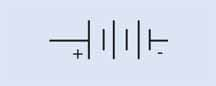open key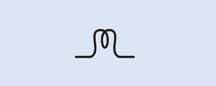cellbulb glows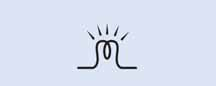battery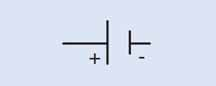bulb does not glow Ans : 1 – D, 2 – E, 3 – A, 4 – C, 5 – B

### V. Arrange in sequenceAns : A DEVICE THAT CONVERTS CHEMICAL ENERGY IN TO ELECTRICAL ENERGY IS CALLED CELL.

### VII. Give very short answer

1. In the given circuit diagram, which of the given switch(s) should be closed. So that only the bulb A glows.Ans :  K1 & K2 keys must be closed.

2. Assertion (A) : It is very easy for our body to receive electric shock.

Reason (R) : Human body is a good conductor of electricity.

1. Both A and R are correct and R is the correct explanation for A.
2. A is correct, but R is not the correct explanation for A.
3. A is wrong but R is correct.
4. Both A and R are correct and R is not the correct explanation for A.

Ans : Both A and R are correct and R is the correct explanation for A.

3. Can you produce electricity from lemon?

Yes, We can produce electricity from lemon.

4. Identify the conductor from the following figures.Ans : Chain

5. What type of circuit is there in a torch light?

simple circuit

6. Circle the odd one out. Give reason for your choice.

Switch, Bulb, Battery, Generator.

Generator (others things are simple circuit materials).

1. Draw the circuit diagram for series connection.2. Can the cell used in the clock gives us an electric shock? Justify your answer.

clock does not give us an electric shock.Because very very low voltage cell is used.

3. Silver is a good conductor but it is not preferred for making electric wires. Why?

More expensive

1. What is the source of electricity? Explain the various power stations in India?

#### Thermal Power station

In thermal power stations, the thermal energy generated by burning coal, diesel or gas is used to produce steam. The steam thus produced is used to rotate the turbine. While the turbine rotates, the coil of wire kept between the electromagnet rotates. Due to electro magnetic induction electricity is produced. Here heat energy is converted into electrical energy.#### Hydel power station

In hydel power stations, the turbine is made to rotate by the flow of water from dams to produce electricity. Here kinetic energy is converted into electrical energy. Hydel stations have long economic lives and low operating cost#### Atomic power station

In atomic power stations, nuclear energy is used to boil water.

The steam thus produced is used to rotate the turbine. As a result, electricity is produced. Atomic power stations are also called as nuclear power stations. Here nuclear energy is converted into mechanical energy and then electrical energy#### Wind mil

In wind mills, wind energy is used to rotate the turbine to produce electricity. Here kinetic energy is converted into electrical energy.2. Tabulate the different components of an electric circuit and their respective symbols.

 Electric component Figure Symbol Remarks Electric cellLonger terminal refers positive and shorter terminal refers negative. Battery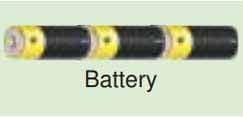Two or more cells connected in series Switch-open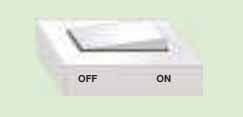Switch is in off position Switch-closed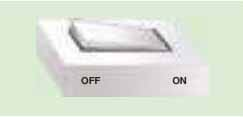Switch is in on position Electric bulbThe bulb does not glowThe bulb glows Connecting wires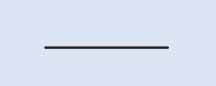Used to connect devices.

3. Write short notes on conductors and insulators.

#### Conductors

The rate of fl ow of electric charges in a circuit is called electric current. The materials which allow electric charges to pass through them are called conductors. Examples: Copper, iron, aluminum, impure water, earth etc.,#### Insulators (Non-Conductors)

The materials which do not allow electric charges to pass through them are called insulators or nonconductors.

Examples: plastic, glass, wood, rubber, china clay, ebonite etc.,